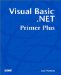# Review Questions

Programmer's TipAs you try the short programs presented in this section, you can type the code into the form's Load() event. You do not need to add text boxes or other controls to the programs. To test the programs, you simply set a breakpoint on the End Sub statement at the end of the form's Load() event. You can, of course, move the cursor over any variable you want to examine while the program is running.

 1: What is the purpose of relational operators? A1: Relational operators are used to compare values. Because relational operators are used to compare two values, they are binary operators that require two operands to do their job. Relational operators are the starting point for making decisions in a program based on the values of data. 2: Suppose a variable named Gender assumes the value for females and 1 for males. How would you write the code that tests the value of Gender and uses a message box to display Male or Female , based on the outcome of the test? A2: The code might look like: ` Dim Gender As Integer Gender = 0 If Gender = 0 Then MessageBox.Show("Female") Else MessageBox.Show("Male") End If ` In this example, you use an If test, by using the relational equality operator, to determine which sex to display, via a call to MessageBox() . 3: What is the value of Result after the following statement is executed? ` Result = 10 > 5 ` A3: You can answer this question by executing the following code: ` Dim Result As Integer Result = 10 > 5 MessageBox.Show(CStr(Result)) ` Before you try running the program, what do you think the value of Result will be, and why? Now run the program and, if the outcome is not what you expected, try to resolve why the answer was different than you expected. (Hint: Visual Basic .NET translates logic True to “1 and logic False to .) 4: What is the value of Result in the following statement? ` Result = (10 > 5) And (5 > 7) ` A4: You can test the answer by using the following code: ` Dim Result As Integer Result = (10 > 5) And (5 > 7) MessageBox.Show(CStr(Result)) ` Is the answer what you expected and, if not, why was your answer wrong? 5: What is the value of Result in the following statement? ` Result = (10 > 5) Or (5 > 7) ` A5: You can test the answer by using the following code: ` Dim Result As Integer Result = (10 > 5) Or (5 > 7) MessageBox.Show(CStr(Result)) ` You should be able to explain the value that is calculated for Result . It is important that you know the difference between this answer and the answer to Review Question 4. 6: What is the value of Result in the following expression? ` Result = Not 3 ` A6: You can test the answer by using the following code: ` Dim Result As Integer i = 3 Result = Not 3 MessageBox.Show(CStr(Result)) ` The outcome of a unary Not operation is the bitwise complement of the expression ”in this case, the value 3 . This is the binary representation of the value 3 : ` 00000011 ` When you determine the bitwise complement of a number, the value of each bit in the expression is flipped , so the binary representation after the Not expression is this: ` 11111100 ` Note that all the 1s become 0s and all the 0s become 1s. However, because the sign bit is now set, the outcome of the expression is viewed as a negative number. Because of the way negative numbers are processed , the answer is “4. 7: Suppose a function named IsItOdd() returns a nonzero result if the number passed to it is odd, and it returns if the number is even. What is displayed in the message box when this program runs? ` Private Sub Form1_Load(ByVal sender As System.Object, ByVal e As _ System.EventArgs) Handles MyBase.Load Dim Result As Integer, TestValue As Integer TestValue = 55 < 100 If IsItOdd(TestValue) Then MessageBox.Show("Hello") Else MessageBox.Show("Good-Bye") End If End Sub Private Function IsItOdd(ByVal num As Integer) As Integer num = num Mod 2 Return num End Function ` A7: The interesting section of code is in the If test. First, the logical test that 55 is less than 100 is logic True , which assigns “1 into TestValue . The call to IsItOdd() passes the “1 to the function, which is an odd (negative) number. The return value, therefore, is “1 . Upon return from the function, the If test examines the return value of “1 and, because any nonzero value is deemed to be logic True , the program displays "Hello" in the message box. 8: The present value of an income stream can be determined by the following equation: ` PV = Income / (1.0 + InterestRate) ^ Years ` For example, if Income is \$100 and the interest rate is 5% and the number of years is 2, the present value is \$90.70. This means that if you put \$90.70 in an asset that yields 5% for 2 years, you would have \$100 in two years. Write the code necessary to calculate the present value of \$200 for 3 years at 5%. A8: Here's the code: ` Dim Value As Double alue = 200 / (1.05) ^ 3 ` The answer is \$172.77. Are the parentheses needed? Why? You might try to generalize this program to use text boxes for the interest rate, the amount, and the number of years, and then display the result in another text box.Visual Basic .NET Primer Plus
ISBN: 0672324857
EAN: 2147483647
Year: 2003
Pages: 238
Authors: Jack Purdum

Similar book on Amazon